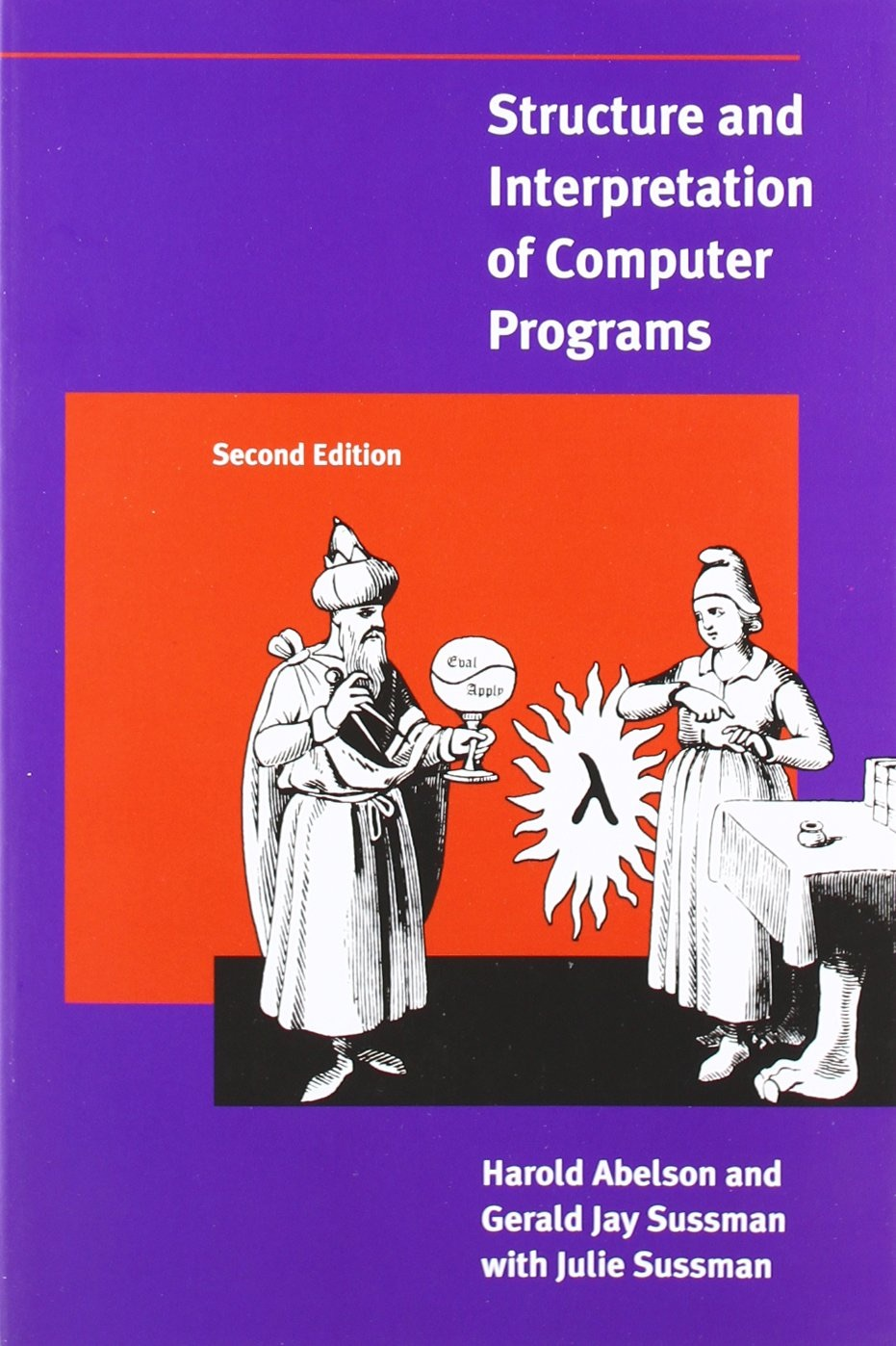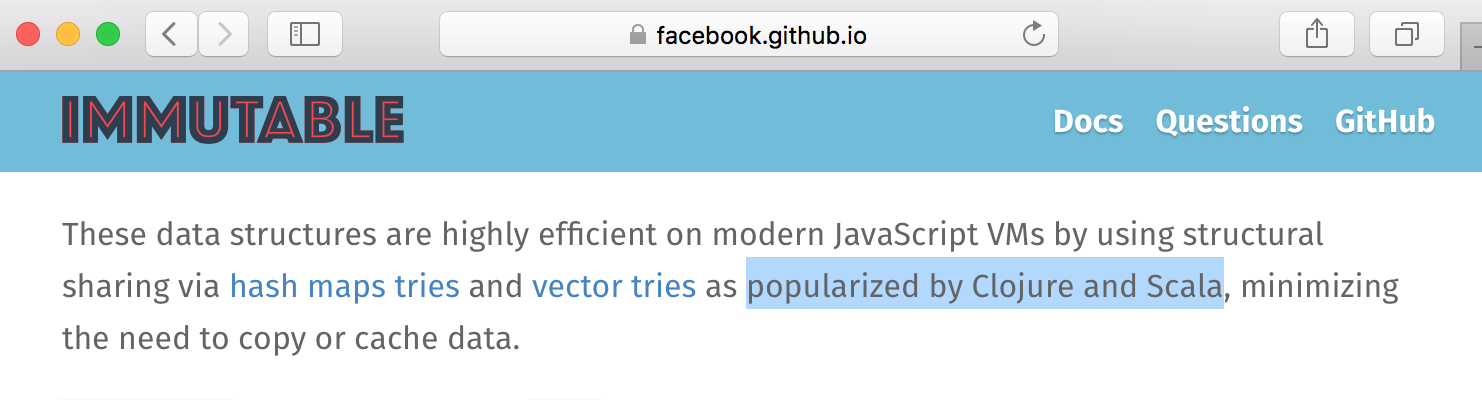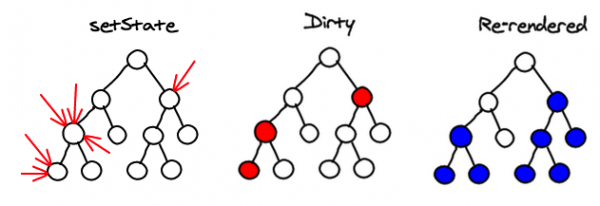## Functional Programming from rich to reachFunctional Programming in Israel
Yehonathan Sharvit

``````
(new Date()).toString()
``````

## Who am I?

• A mathematician

• A pragmatic theorist

• A coder

• A freak of interactivity

• Barclays - head of functional programming

• (We're hiring...)

• Founded a Audiology Startup with 30K LOCs in Clojurescript

• Author of KLIPSE## Agenda

• Functional programming - immutability

• React.js - a functional frontend framework

• Interludes

• JSX - a "macro" system

• ClojureScript - LISP on top of javascript

• Code interactivity everywhere with KLIPSE## Functional Programming - Immutability

In non-functional programming, the default API mutates our objects

``````
const a = {size: 42};
b = a;b.size = 43
a
``````

In functional programming e.g. clojure, the default API doesn't mutate our objects

``````
(def a {:size 42})
(def b (assoc a :size 43))
a
``````

EcmaScript 6 got inspired by functional programming - and propses an immutable API:

``````
const a = {size: 42};
b = Object.assign({}, a); b.size = 43
a
``````

Does it work as expected?

``````
const a = {size: {shoes: 42}};
b = Object.assign({}, a)
b.size.shoes = 43
a
``````

## Functional Programming - Immutable.js``` !!Immutable ```
``````
a = Immutable.fromJS({size: {shoes: 42 }});
b = a.setIn(["size", "shoes"], 43)
a
``````

## React.js: the functional frontend framework

The principles

1. Virtual DOM

2. Immutability

3. Pure functionsDespite the fact that `React.js` is functional, it is popular!

## Live Coding - in the browser

### Tic Tac Toe## React - The basics

Let's load react javascript files from cdnjs.com:

``````
!!React
``````

## Interlude - JSX

JSX is ...

kind of a macro system.

``````
const element = <h1>Hello, world!</h1>;
``````
``````
const element = <h3 style= {{color:"red"} }>Hello, world -- 14*3={14*3}!</h3>;
ReactDOM.render(element, container);
``````

The JSX transpiler runs before the code is deployed.
It's a compile time syntactic sugar.
Compare vs. angular.js

## Tic Tac Toe - The square

Square is a pure component

``````
``````
``````
ReactDOM.render(React.createElement(Square, {value: 42,
``````

## Tic Tac Toe - The board

`Board` is a full react component with `constructor`, `render` and a couple of helper functions

``````
ReactDOM.render(React.createElement(Board, {}), container);
``````

## Interlude - Clojurescript

How do we decide who is the winner of the game?

There are only 8 possibilities to fill a row in the board

``````
(ns my.game)
(defn calculate-winner [squares]
(let [lines [[0 1 2] [3 4 5] [6 7 8] [0 3 6] [1 4 7] [2 5 8] [0 4 8] [2 4 6]]]
(->> (map (fn [[a b c]] (and (some? (get squares a))
(= (get squares a) (get squares b) (get squares c))
(get squares a)))
lines)
(filter identity)
first)))
``````
``````
(require '[cljs.test :refer [run-tests] :refer-macros [deftest testing is are]])
``````
``````
(deftest calc-winner
(testing "basic"
(is (= (calculate-winner ["X" "X" "X"]) "X"))
(is (= (calculate-winner ["X" "X" "O"]) nil))
(is (= (calculate-winner ["O" "O" "O"]) "O"))))

(run-tests)
``````
``````
(deftest calc-winner
(are [in out] (= (calculate-winner in) out)
["X" "X" "X"] "X"
["X" "O" nil
"X" "O" nil
"X" nil nil] "X")))

(run-tests)
``````

## Tic Tac Toe - with players

``````
ReactDOM.render(React.createElement(BoardWithPlayers, {}), container);
``````

## Summary

• Functional programming influence on main stream technologies

• EcmaScript6

• Immutable.js

• React.js

• ClojureScript

• Live coding in the browser with klipse

## Questions?/